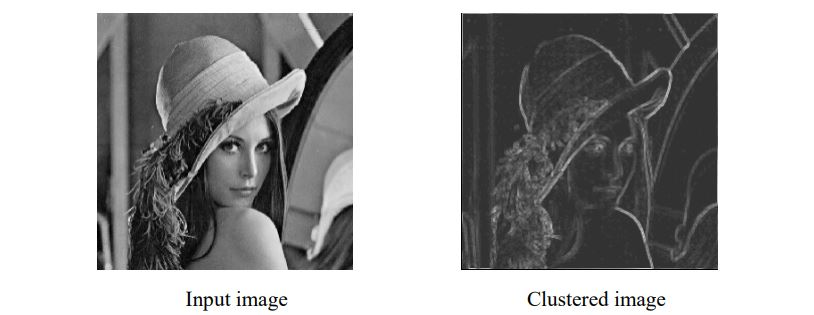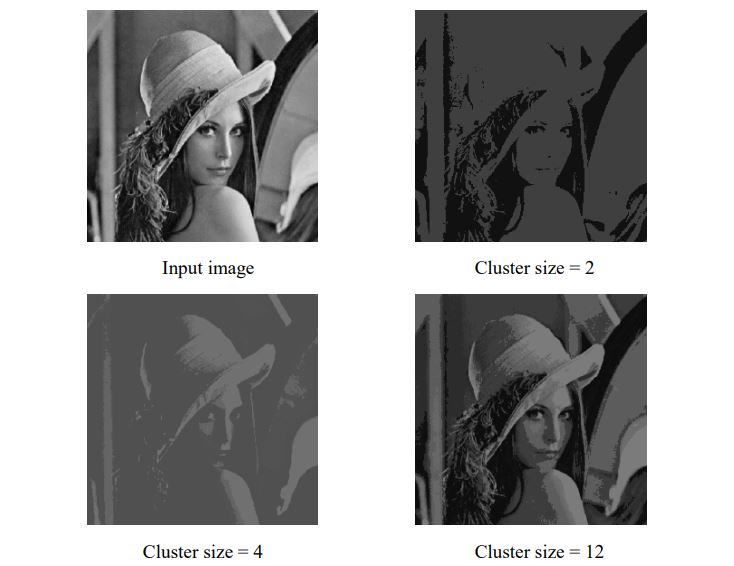DBSCAN - Digital Image Processing

Final Year Project, Burdwan University

Hi, welcome to a report on my final year project on digital image processing at University of Burdwan. If you’re already familiar with the subject, you might want to peek into our work here.

After months of working out on ideas under our mentor, we looked into developing a novel algorithm for performing Density-based spatial clustering of applications with noise (DBSCAN) for image clustering.

DBSCAN is a data clustering algorithm proposed by Martin Ester, Hans-Peter Kriegel, Jörg Sander and Xiaowei Xu in 1996. It is a density-based clustering algorithm which finds a number of clusters starting from the estimated density distribution of corresponding nodes. The definition of DBSCAN is a cluster based on the notion of density reachability.

Novel methodology for DBSCAN

Through the project, we proposed a novel algorithm by modifying the existing DBSCAN algorithm for image processing. We devised an algorithm which will make DBSCAN insensitive to clustering parameters (MinPts and Eps). Moreover, it allows us to handle image data with varying densities with ease. The proposed algorithm works as follows:

• An image (.bmp format) of interest is taken and gray levels $(\alpha)$ are derived from image and pixel matrix is prepared.
• $8-$Neighbour distance of each pixel is found out considering every pixel as center pixel.
• Maximum distance for each pixel is computed.
• Dividing the number of pixels/points by maximum distance gives density of the center pixel.
• If a pixel falls in varying density region, the pixel is assigned to the highest density region and the center pixel corresponding to the highest density region is considered to be center-point of the region.
• Now, a histogram of density values is plotted on $XY$ plane with $X$ as the gray values and $Y$ as number of pixels.
• Alternatively, a clustered image can also be developed using the known density regions.

The varying density regions represent varying clusters with center points for each cluster as determined in previous steps.

$Distance\ of\ P\ w.r.t.\ Q\ ;\ {D}_{i}(P,Q)\ = \lvert{u-v}\rvert$

Where, $P$ is a pixel with $\alpha\ = u$

$\qquad\ Q$ is a pixel with $\alpha\ = v$

$8-Neighbour\ distance\ of\ a\ pixel\ P\ = max({D}_{1} (P,Q), {D}_{2}(P,Q), …, {D}_{n}(P,Q))$

$Density\ of\ Pixel\ (P) = \dfrac{Number\ of\ pixels\ with\ \alpha\ >\ 0}{{N}_{8}(P)\ distance}$

Cons of conventional method of DBSCAN

• The process cannot be partitioned for multiprocessor systems.
• Datasets with altering densities are tricky.
• Sensitive to clustering parameters, MinPts and Eps.
• Fails to identify clusters if density varies and if the dataset is too sparse.
• Sampling affects density measure.

Our algorithm’s advantage over existing algorithm

Using our proposed algorithm, we are able to eliminate the following demerits of conventional DBSCAN.

Results from our algorithmHere, we find that the novel DBSCAN algorithm is successfully able to create clusters in the input image without using any parameters which can alter the results otherwise. This is useful as this algorithm is not sensitive to any parameters. Also, there are no random initial points as in KMeans clustering which makes the algorithm return consistent results.Fig: KMeans clustering results for comparison

The errors in determining regions of varying densities are eliminated in the novel algorithm of DBSCAN as the algorithm correctly clusters the edges differently as the regions of high varying density. The overall image appears gray because, most of the image is illuminated with similar gray value and hence the dark regions denote another cluster. Also, there are some gray intensity regions in the clustered image which relates to hair and noise.

Project MembersBiswajit Roy
Systems Engineer

My research interests include digital image processing, cloud computing, distributed systems and pipelines.